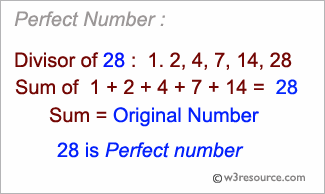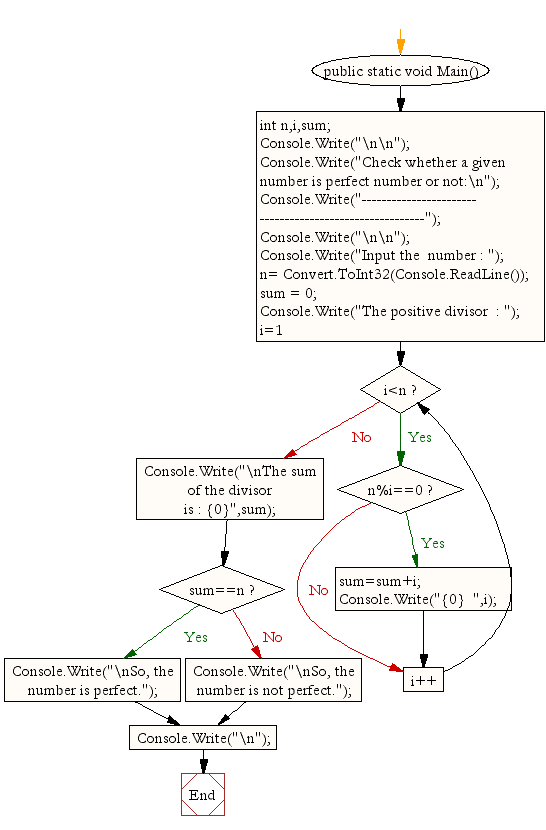﻿ C# - Check whether a given number is perfect number or not# C# Sharp Exercises: Check whether a given number is perfect number or not

## C# Sharp For Loop: Exercise-27 with Solution

Write a C# Sharp Program to check whether a given number is perfect number or not.Sample Solution:-

C# Sharp Code:

``````/*Perfect number is a positive number which sum of all positive divisors excluding that number is equal to that number. For example 6 is perfect number since divisor of 6 are 1, 2 and 3.  Sum of its divisor is 1 + 2+ 3 = 6*/
using System;
public class Exercise27
{
public static void Main()
{
int n,i,sum;

Console.Write("\n\n");
Console.Write("Check whether a given number is perfect number or not:\n");
Console.Write("--------------------------------------------------------");
Console.Write("\n\n");

Console.Write("Input the  number : ");
sum = 0;
Console.Write("The positive divisor  : ");
for (i=1;i<n;i++)
{
if(n%i==0)
{
sum=sum+i;
Console.Write("{0}  ",i);
}
}
Console.Write("\nThe sum of the divisor is : {0}",sum);
if(sum==n)
Console.Write("\nSo, the number is perfect.");
else
Console.Write("\nSo, the number is not perfect.");
Console.Write("\n");
}
}
```
```

Sample Output:

```Check whether a given number is perfect number or not:
--------------------------------------------------------
Input the  number : 20
The positive divisor  : 1  2  4  5  10
The sum of the divisor is : 22
So, the number is not perfect.
```

Flowchart:C# Sharp Code Editor:

Contribute your code and comments through Disqus.

What is the difficulty level of this exercise?

Test your Programming skills with w3resource's quiz.

﻿